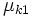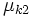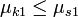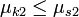# Pulley system for block piled on block on an inclined plane

This article discusses a scenario/arrangement whose statics/dynamics/kinematics can be understood using the ideas of classical mechanics.
View other mechanics scenarios

## The scenario

Consider one block placed on top of another, with the bottom block being$A$ and the block on top being$B$.$A$ is on a fixed inclined plane making an angle of$\theta$ with the horizontal and the surface of contact between$A$ and$B$ is parallel to the inclined plane, i.e., that surface also makes an angle of$\theta$ with the horizontal. There is a pulley system connecting$A$ and$B$, with both sides of the pulley rope being parallel to the inclined plane.

Consider the following conventions:

•$\mu_{s1}$ is the limiting coefficient of static friction between$A$ and the inclined plane.
•$\mu_{k1}$ is the coefficient of kinetic friction between$A$ and the inclined plane.
•$\mu_{s2}$ is the limiting coefficient of static friction between$A$ and$B$.
•$\mu_{k2}$ is the coefficient of kinetic friction between$A$ and$B$.

Assume that$\mu_{k1} \le \mu_{s1}$ and$\mu_{k2} \le \mu_{s2}$.

We assume the pulley and string to be massless, so that the tension at both ends of the string is equal.